AUTOGAMMA

 Modifies an image to automatically apply a gamma correction.

 USAGE: autogamma [-c colormode] infile outfile USAGE: autogamma [-h or -help] -c .... colormode ..... colorspace/channel to use to compute ....................... gamma statistics; choices are: gray, ....................... intensity, luminance, lightness, brightness, ....................... average, magnitude, rgb; default=luminance -m .... midrange ...... midrange value from which to compute gamma; ....................... 0

EXAMPLES

 Colormode Results On Dark Image Original Image Arguments: -c rgbArguments: -c luminance Arguments: -c gray (or intensity) Arguments: -c average Arguments: -c lightness Arguments: -c magnitude Arguments: -c brightnessThe Following Images Were Obtained At http://images.google.com/

 Original Image Arguments: -c luminanceOriginal Image Arguments: -c luminance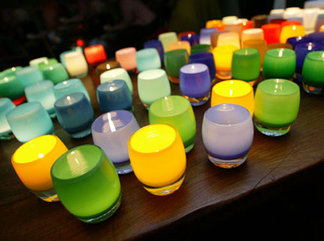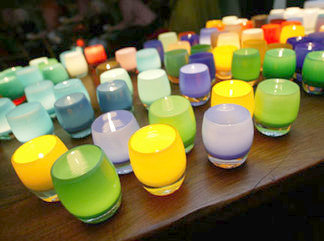Original Image Arguments: -c luminanceOriginal Image Arguments: -c luminance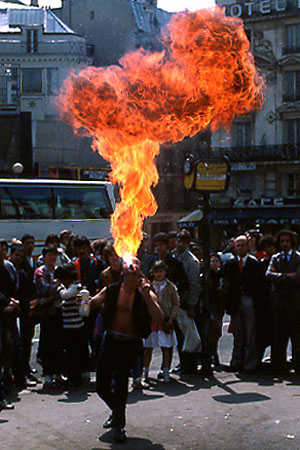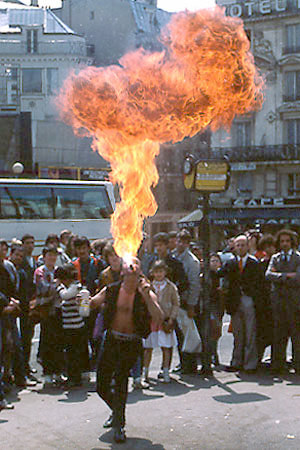Original Image Arguments: -c luminanceOriginal Image Arguments: -c luminance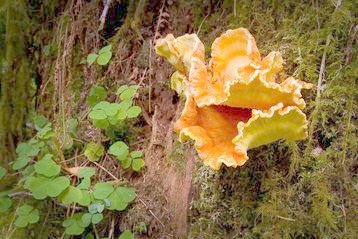Original Image Arguments: -c luminanceWhat the script does is as follows: Converts the image to RGB Generates a copy of the image in the desired colorspace/channel Accesses the mean statistics for this image Computes a gamma value from the mean Passes the gamma value to -gamma and applies to each channel of the RGB image This is equivalent to the following IM commands for the luminosity colormode. convert \$infile -colorspace RGB \$tmp1 convert \$tmp1 -colorspace Rec709Luma \$tmp2 mean=`convert \$tmp2 -format "%[mean]" info:` mean=`convert xc: -format "%[fx:100*\$mean/quantumrange]" info:` gammaval=`convert xc: -format "%[fx:log(\$mean/100)/log(\$midrange)]" info:` convert \$tmp1 -gamma \${gammaval} \$outfile